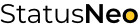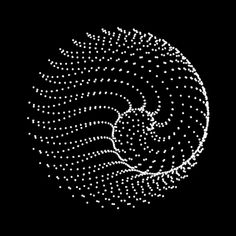WE ARE EXPERTS IN TECHNOLOGY

## Let’s Work Together# StatusNeo## Naive Bayes – the genesis algorithm

Do you ever wonder from where the concept of self-learning machines originated? Exactly when and which scientist figured out a way to model the ‘learning’ mathematically? Today many people talk about artificial intelligence but which algorithm gave rise to all the possibilities? Well.. let’s see!

Let’s understand. We know conditional probability :

The above equation means the probability of event A, given that event B has already occurred is equal to the probability of occurrence of both A and B divided by the probability of B.

Similarly, we can write P(B/A) as:

Let’s just for a second visualize the above equation as:

<== Let’s put this value of this term in the initial equation:

This equation above is Baye’s rule.

1. P(A|B) is called Posterior probability
2. P(A) is called Prior probability
3. P(B) is called Normalizing probability
4. P(B|A) is called Likelihood probability

Generally in Naive Bayes, these possibilities for event B are possible.

1. B is UNIVARIATE

• B is Discrete
• Binomial
• Multinomial
• B is continous

2. B is MULTI-VARIATE

• all features in B are continuous
• all features in B are discrete – binomial or multinomial
• some features in B are discrete and some are continuous

Now, why did I discuss all these details? I want you to know that this Naive Bayes algorithm lays the foundation for logistics regression, a fundamental classification algorithm even used in neural networks. Not only in logistics regression but linear regression, dimensionality reduction algorithm like LDA and so many other advanced algorithms. How? Let’s first see for logistic regression and that too for binary classification.

Let’s say our data has two classes `1` and `0`. And, considering that our data is independent P(A) = P(B) = 0.5 in both cases. Hence,

So,

We can say Binary Logistic Regression is a discriminative classifier, because here, instead of first calculating likelihood probability and the calculating posterior probability, we will directly calculate the posterior probability by making a PDF(probability density function) for posterior probability.

### Let’s see how:

We know, that our posterior probability, for each example in our data(rows), for one of the classes (if our data has only 2 classes) looks like what we are considering from the perspective of PDF:

And, for another class:

Suppose: our data(considering preprocessed and normalized data) has N rows(examples) and M columns(features)

1. For each example `i` in all `N` examples, there will be an actual class that it must belong to. That class will be either `0` or `1` (in encoded form).

2. Let’s see what the class column will look like:

3. Now what we are going to make a PDF of the posterior probability is that we are going to combine the posterior probability of each class in a likelihood function. Let’s see how:

A. For each example `i`, posterior probability can be written as :

B. Combining the above formula for all examples :

C. Taking both sides `log` with base `e`, our Likelihood Function becomes:

This is our final Log-Likelihood Function, which we have to maximize, i.e:

To dissolve the negative sign outside our optimization problem, we take it inside our log-likelihood function and our new likelihood function and optimization problem become:

Now, this minimization further can be implemented using Gradient Descent or another optimizer.

That is how Naive Bayes laid the foundation for binary logistic regression.

Similarly, in the upcoming blogs, we will see how it also makes its way into multi-class classification, linear regression, and even neural networks.

A question still remains- From where did the concept of self-learning machines originate?

How did learning about Naive Bayes concepts help ? Well, to answer that question- we are trying to calculate a probability of a certain event such that somethings have already taken place in past, now if we just twist our perception a little, is it not like self learning we humans do? What do you think?